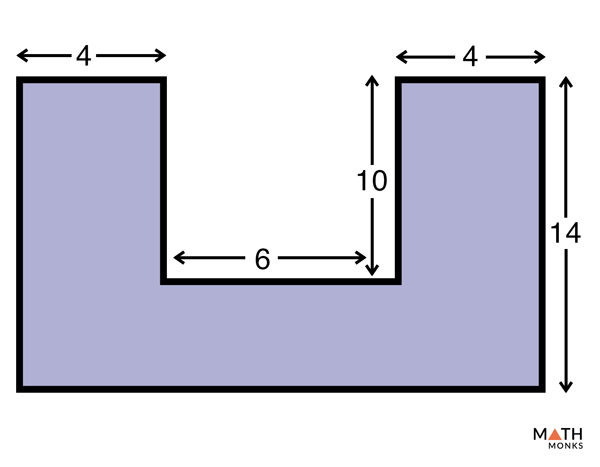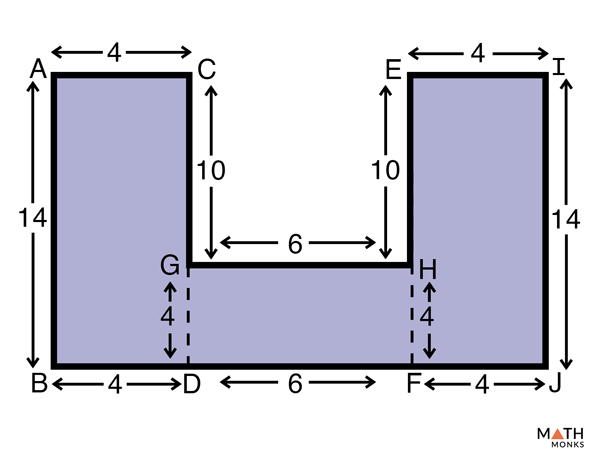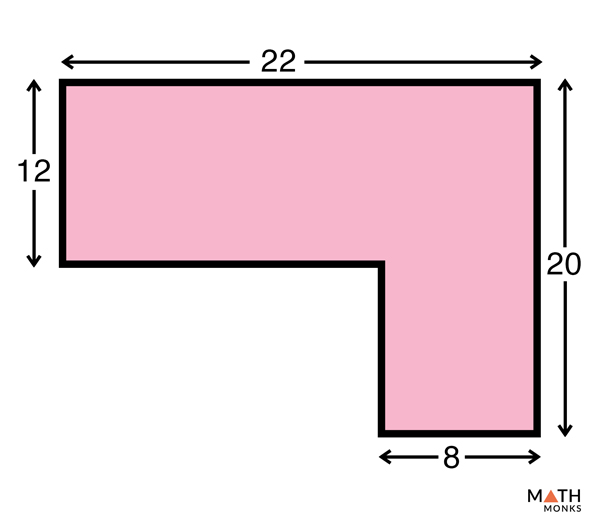# Convex and Concave Polygons

Based on their interior angles, all polygons are broadly classified into two groups: convex and concave.

## What is a Convex Polygon

A convex polygon is a polygon that has all its interior angles less than 180°. All the diagonals of a convex polygon lie inside the closed figure. A convex polygon can be both regular and irregular. Regular convex polygons have all sides of the same length and all interior angles of the same measure (less than 180°). Convex polygons that are not regular are called irregular convex polygons.

Examples: Triangles, all convex quadrilaterals, regular pentagon, and regular hexagon are all convex polygons. A square is a regular convex polygon.

### Properties

1. All interior angles measure less than 180°; ∠ABC, ∠BCD, ∠CDE, ∠DEF, ∠EFA, and ∠FAB measure less than 180°
2. All the nine diagonals lie inside the closed figure; diagonals AC, AD, AE, BD, BE, BF, CE, CF, and CA lie inside the figure.
3. Sum of all the exterior angles measure 360°
4. Sum of all the interior angles having n-sides is given by (n – 2) x 180°

In the given pentagon ABCDE all interior angles are of equal measure. State whether it is a convex polygon.

Solution:

As we know,
Interior angle of a Regular Polygon = (n-2) × 180° /n, here n = 5
= (5-2) x 180° /5
= 3 x 180° /5
= 108°
Since all the five interior angles in the given pentagon measure less than 180°, it is a convex polygon.

### Formulas

#### Area

It is the total space enclosed within the polygon. The formula is given below:

Area (A) = ½|(x1y2 – x2y1)+(x2y3– x3y2)+……..+(xny1– x1yn)|, here (x1, y1), (x2, y2), (x3, y3),…….., (xn, yn) are the vertices of the polygon on the coordinate plane

Find the area of a convex polygon with vertices (5, 2) (12, 8) and (5, -2)

Solution:

As we know,
Area (A) = ½|(x1y2 – x2y1)+(x2y3– x3y2)+(x3y1 – x1y3)|,
here (x1, y1) = (5, 2), (x2, y2) = (12, 8), (x3, y3) = (5, -2)
= ½|(40-24)+ (-24-40)+ (10)-10)|
= ½|16-64|
= 48/2

#### Interior Angles

The interior angles of a polygon are the angles found inside the polygon. A polygon has the same number of interior angles as the number of sides.

Sum of the Interior Angles

It is the total measure of all the interior angles combined in the polygon. The formula to determine the sum of all angles in any convex regular polygon is given below:

Sum of the measure of interior angles = (n-2) × 180°, here n = total number of sides of the polygon

Calculate the sum of the interior angles in a 14-sided convex regular polygon.

Solution:

As we know,
Sum of the measure of interior angles = (n-2) × 180°, here n = 22
= (14-2) x 180°
= 2160°

One Interior Angle

Each interior angle measures the same in a regular convex polygon and can be obtained by dividing the sum of the interior angles by the total number of sides. The formula is given below:

Interior angle of a Convex Polygon = (n-2) × 180° /n, here n = total number of sides of the polygon

Find the measure of the interior angles of a convex regular polygon having 12 sides.

Solution:

As we know,
Interior angle of a Regular Polygon = (n-2) × 180° /n, here n = 18
= (18-2) x 180° /18
= 16 x 180° /18
= 160°

#### Exterior Angles

The exterior angle of a polygon is the angle formed by one side and the extension of the adjacent side.

The exterior angle sum theorem states that the sum of the exterior angles of any convex polygon is 360°. For regular convex polygons having n sides, each exterior angle is determined using the following formula:

Exterior angle of a Convex Regular Polygon = 360°/n, here n = total number of sides of the polygon

Calculate the exterior angle in a 15-sided regular convex polygon.

Solution:

As we know,
Exterior angle of a Regular Polygon = 360°/n, here n =15
= 360°/15
= 24°

## What is a Concave Polygon

A concave polygon, also known as a non-convex polygon, has at least one of its interior angles measuring more than 180°. Some diagonals of a concave polygon lie outside the closed figure.

All concave polygons are irregular since all the interior angles are of different measures. Thus concave polygons are never regular.

Examples: A dart or an arrowhead in quadrilaterals, some irregular pentagon, and hexagon

### Properties

1. Has at least one reflex angle that measures greater than 180° and less than 360°; ∠DEF is greater than 180° and less than 360°
2. Has at least one vertex pointing inwards; vertex C point inwards
3. Has one or more diagonals that lie outside the closed figure; diagonal DF lies outside the closed figure
4. If a line segment is drawn crossing the concave polygon, it will intersect the boundary more than twice

The sum of interior angles of the given hexagon ABCDEF is 720°. If ∠ABC = 78°, ∠BCD = 140°, ∠CDE = 80°, ∠EFA =88°, ∠FAB = 130°. Find ∠DEF and state whether the polygon ABCDEF is concave.

Solution:

As we know,
The sum of the angles in hexagon ABCDEF = 720°
So, ∠ABC + ∠BCD + ∠CDE +∠DEF + ∠EFA + ∠FAB =
78° + 140° + 80° +∠DEF + 88° + 130° = 720°
=> ∠DEF = 720° – 516°
=> ∠DEF = 204°
Since ∠DEF is greater than 180°, polygon ABCDEF is a concave polygon. Also if we draw a line joining points D and F, we find that the diagonal DF lies outside the closed figure, which further proves that ABCDEF is a concave polygon.

### Formulas

#### Area

Since all the sides and interior angles of a concave polygon are not equal there is no standard formula to determine their area. To determine the area of a concave polygon, we have to split the polygon into shapes such as triangle, rectangle, parallelogram, or other shapes, find the area of each such shapes, and finally adding them to get the total area of the polygon. Thus the area of a concave polygon is:

Area (A) = Sum of the area of all the shapes available within the polygon

#### Perimeter

It is the total distance covered around the boundary of the polygon. The perimeter of a concave polygon is thus obtained by simply adding together the length of all sides. Thus the perimeter is:

Perimeter (P) = Sum of all the sides of the polygon

Let’s understand these concepts using examples.Find the area and perimeter of the concave polygon with the given side measurements.Solution:

The given polygon is divided into three rectangles. Finding the area of each rectangle and adding them will give the area of the polygon.
Thus, area of the polygon = Area of rectangle ABCD + Area of rectangle GDHF + Area of rectangle EFIJ
= (14 x 4) + (6 x 4) + (14 x 4)
= 56 + 24 + 56
= 136 square units
Perimeter of the polygon = AB + BD + DF +FJ + IJ+ EI + EH + GH + CG + AC
= (14 + 4 + 6 + 4 +14 + 4 +10 + 6 +10+ 4)
= 76 unitsFind all the sides of the given concave polygon. Then find its area and perimeter. (Trick: Divide the given irregular polygon into two regular polygons)Solution:

The given polygon is divided into one rectangle and one square. Finding the area of the rectangle and the square and adding them will give the area of the polygon.
Thus, area of the polygon = Area of rectangle ABGF + Area of square CDEG
= (22 x 12) + (8x 8)
= 264 + 64
= 328 square units
Perimeter of the polygon = AB + BC + CD + DE + FE + AF
= (12 + 14 + 8 + 8 + 20 + 22)
= 84 units

#### Interior Angles

To determine the sum of the interior angles we use the following formula:

Sum of interior angles = (n-2) × 180°, here n = total number of sides of the polygon

#### Exterior Angles

Like all other polygons, the exterior angle sum theorem stating that all exterior angles add up to 360° is true for all concave polygons.

## FAQs

Q1. How many sides does a convex polygon have?

Ans. A polygon should have at least three sides and can have any maximum number of sides.

Q2. Why an equiangular triangle is a regular convex polygon?

Ans. An equiangular triangle has all three sides of equal length and all angles of equal measure. Since the sum of the angles in a triangle is 180° each of the angles measure 60°. Thus an equiangular triangle is always a regular convex polygon.

Q3. Name the line segment that joins two nonconsecutive vertices of a convex polygon?

Ans. Diagonal is the line segment that joins two nonconsecutive vertices of a convex polygon